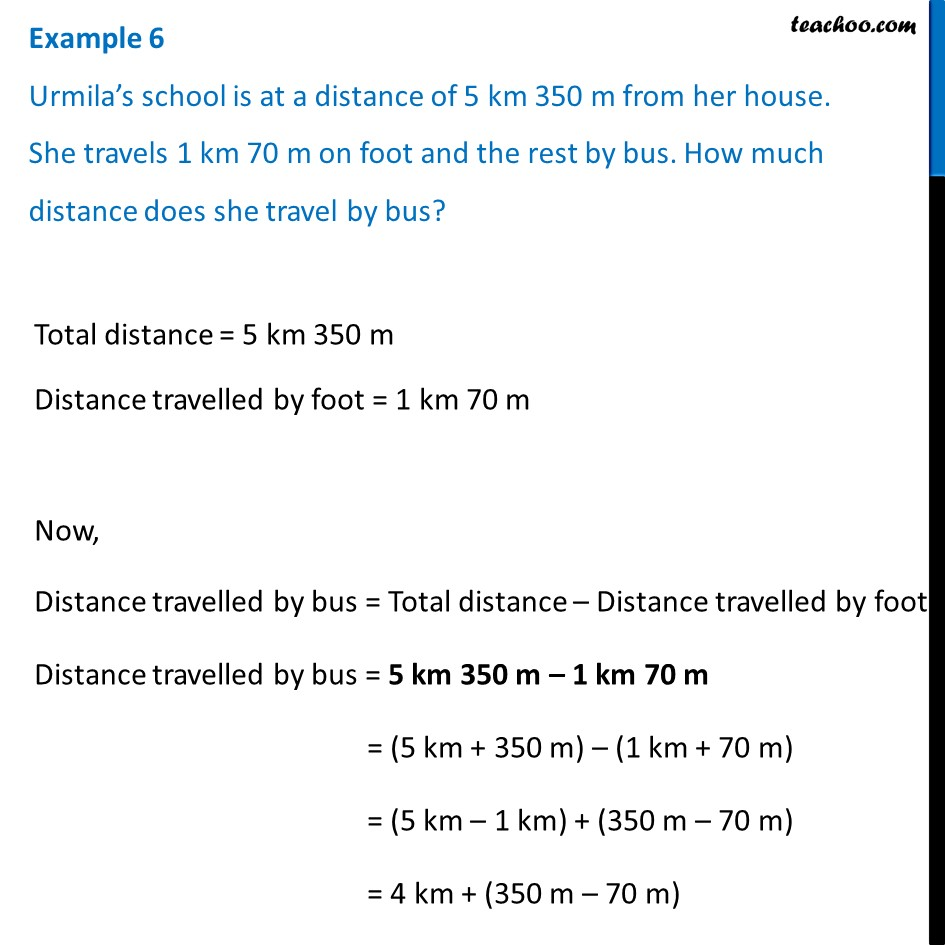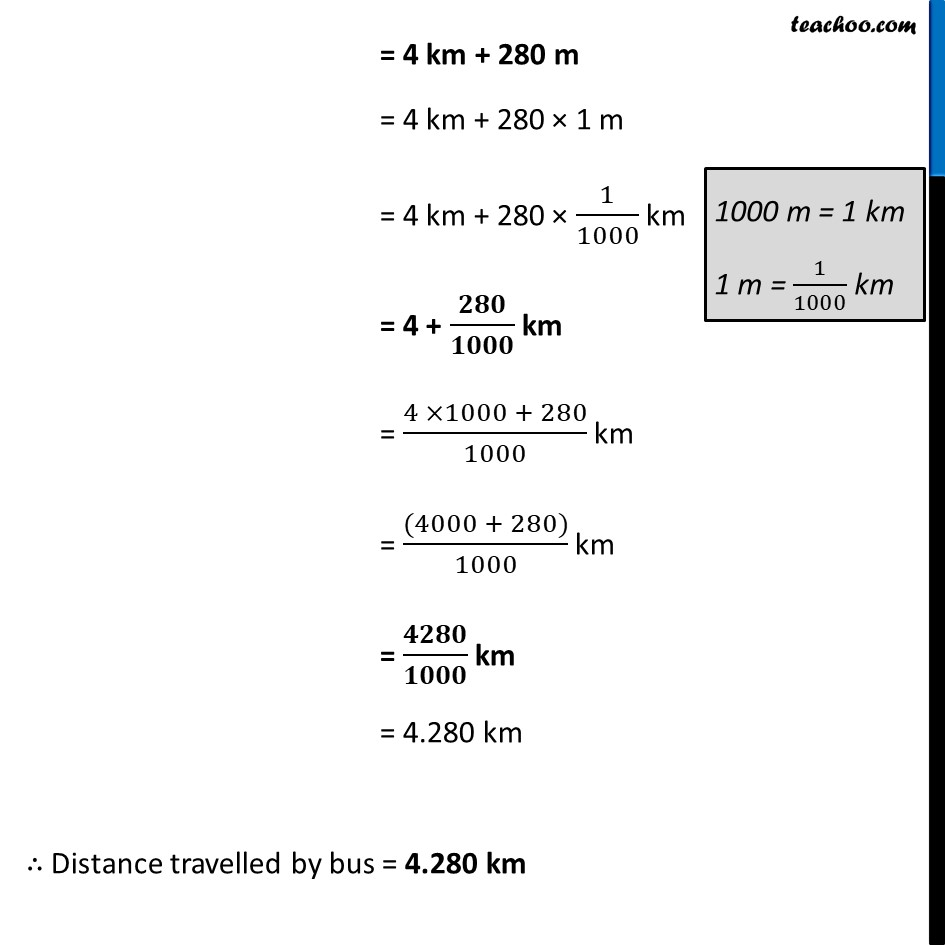Examples

Chapter 8 Class 6 Decimals
Serial order wiseLearn in your speed, with individual attention - Teachoo Maths 1-on-1 Class

### Transcript

Example 6 Urmila’s school is at a distance of 5 km 350 m from her house. She travels 1 km 70 m on foot and the rest by bus. How much distance does she travel by bus? Total distance = 5 km 350 m Distance travelled by foot = 1 km 70 m Now, Distance travelled by bus = Total distance – Distance travelled by foot Distance travelled by bus = 5 km 350 m – 1 km 70 m = (5 km + 350 m) – (1 km + 70 m) = (5 km – 1 km) + (350 m – 70 m) = 4 km + (350 m – 70 m) = 4 km + 280 m = 4 km + 280 × 1 m = 4 km + 280 × 1/1000 km = 4 + 𝟐𝟖𝟎/𝟏𝟎𝟎𝟎 km = (4 ×1000 + 280)/1000 km = ((4000 + 280))/1000 km = 𝟒𝟐𝟖𝟎/𝟏𝟎𝟎𝟎 km = 4.280 km ∴ Distance travelled by bus = 4.280 km